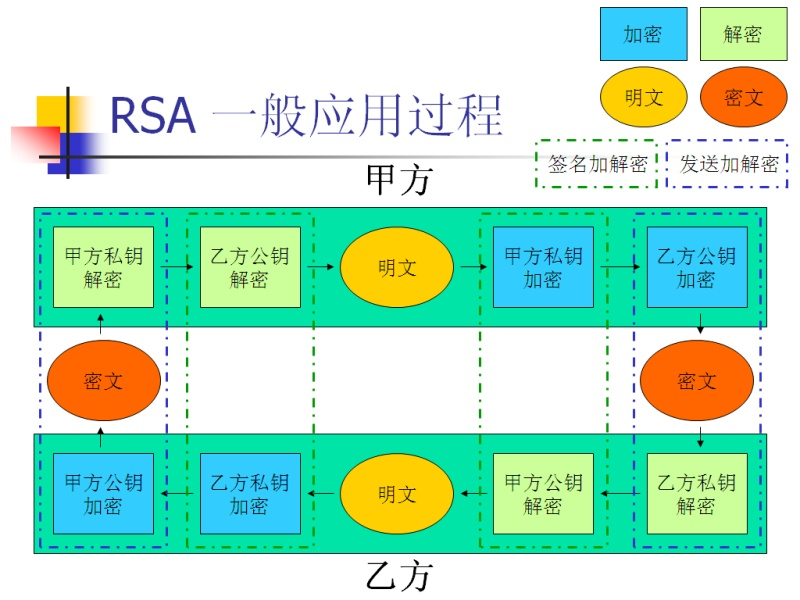• 可以运行的C++版RSA加密解密算法，有注释详细
• RSA加密解密算法，相当不错的例子，适合初学者参考
• rsa加密解密算法C语言代码#include#include#include #include #include #include #define MAX 100#define LEN sizeof(struct slink)void sub(int a[MAX],int b[MAX] ,int c[MAX] );struct slink{ int bignum[MAX];/*...
rsa加密解密算法C语言代码#include#include#include    #include   #include #include #define MAX 100#define LEN sizeof(struct slink)void sub(int a[MAX],int b[MAX] ,int c[MAX] );struct slink{ int  bignum[MAX];/*bignum用来标记正负号，1正，0负bignum来标记实际长度*/    struct slink *next;};/*/--------------------------------------自己建立的大数运算库-------------------------------------*/void  print(  int a[MAX] )   {   int i;   for(i=0;iint  cmp(int a1[MAX],int a2[MAX]){   int l1, l2;int i;l1=a1;l2=a2;if (l1>l2)     return 1;    if (l1=0;i--)   {   if (a1[i]>a2[i])   return 1 ;   if (a1[i]void mov(int a[MAX],int *b){int j;for(j=0;jvoid mul(int a1[MAX],int a2[MAX],int *c){ int i,j; int y; int x; int z; int w; int l1, l2;l1=a1[MAX-1];l2=a2[MAX-1];if (a1[MAX-2]=='-'&& a2[MAX-2]=='-')c[MAX-2]=0;else if (a1[MAX-2]=='-')c[MAX-2]='-';else if (a2[MAX-2]=='-')c[MAX-2]='-'; for(i=0;ivoid add(int a1[MAX],int a2[MAX],int *c){int i,l1,l2; int len,temp[MAX]; int k=0;l1=a1[MAX-1];l2=a2[MAX-1];if((a1[MAX-2]=='-')&&(a2[MAX-2]=='-')){c[MAX-2]='-';}else if (a1[MAX-2]=='-'){mov(a1,temp);temp[MAX-2]=0;sub(a2,temp,c);return;}else if (a2[MAX-2]=='-'){mov(a2,temp);temp=0;sub(a1,temp,c);return;}if(l1len) {  for(i=len;i
展开全文• RSA 加密解密算法 1.RSA 算法的基本概念 2.RSA 加密算法实现 3.RSA 解密算法实现 4. 遇到的问题 5. 总结 RSA 加密解密算法基本概念 RSA 公钥加密算法是 1977 年由 Ron Rivest Adi Shamirh 和 LenA dleman 开发的 RSA...
• 主要介绍了php的RSA加密解密算法原理与用法,结合实例形式分析了rsa加密解密算法的相关概念、原理及PHP使用RSA加密解密算法的具体实现技巧,需要的朋友可以参考下
• RSA混合密码系统.vc++ RSA加解密算法,visual c++
• 网上找不到DELPHI版RSA加解密算法，只能自己写，XE3下实测通过，结果正确。 .TXT后缀名请改成.PAS即可。公钥、私钥请自己生成。
• 主要介绍了Java实现的RSA加密解密算法,结合实例形式分析了java RAS加密解密算法的相关实现技巧,需要的朋友可以参考下
• 与DES不同，RSA算法中，每个通信主体都有两个钥匙，一个公钥一个私钥。就是有2把钥匙1。使用publicKey可以对数据进行加密2。使用Key才能对数据进行解密单方向传输用公钥加密的数据，只有私钥能解开（可用于加密）；...


与DES不同，RSA算法中，每个通信主体都有两个钥匙，一个公钥一个私钥。就是有2把钥匙1。使用publicKey可以对数据进行加密2。使用Key才能对数据进行解密单方向传输用公钥加密的数据，只有私钥能解开（可用于加密）；同时，使用私钥加密的数据，只有公钥能解开（签名）。但是速度很慢（比私钥加密慢100到1000倍），公钥的主要算法有RSA，还包括Blowfish,Diffie-Helman等 公钥与私钥1.权威数字认证机构（CA）给所有通信主体（个人或组织）颁发公钥和私钥，彼此配对，分别唯一。2.私钥好比数字指纹，同时具有解密和加密功能。个人保管，不公开。3.公钥好比安全性极高的挂号信箱地址，公开。
公私钥加解密举例设若甲有一份需保密的数字商业合同发给乙签署。经过如下步骤： 1. 甲用乙的公钥对合同加密。 2. 密文从甲发送到乙。 3. 乙收到密文，并用自己的私钥对其解密。 4. 解密正确，经阅读，乙用自己的私钥对合同进行签署。 5. 乙用甲的公钥对已经签署的合同进行加密。 6. 乙将密文发给甲。 7. 甲用自己的私钥将已签署合同解密。 8. 解密正确，确认签署。
公私钥加解密说明从以上步骤，我们知道： 1. 用公钥加密的密文能且只能用与其唯一配对的私钥才能解开。 2. 如果某份密文被解开，那么肯定是密文的目标信息主体解开的。 3. 私钥因其唯一标识所有者的属性，被用于数字签名，具有法律效力。
一。 公私钥生成1.随机选定两个大素数p, q.2.计算公钥和私钥的公共模数 n = pq .3.计算模数n的欧拉函数 φ(n) .4.选定一个正整数e, 使1 < e < φ(n) , 且e与φ(n)互质.5.计算d, 满足 de ≡ 1  (mod φ(n) ), (k为某个正整数).6.n与e决定公钥, n与d决定私钥.二。加解密该过程为小张给小李发消息，公钥为小李的公钥(n & e), 私钥为小李的私钥(n & d).1.小张欲给小李发一个消息M, 他先把M转换为一个大数m < n, 然后用小李的公钥(n & e)把m加密为另一个大数:   c = me    mod n2.小李收到小张发来的大数c, 着手解密. 通过自己的私钥(n & d), 得到原来的大数m:   m = cd    mod n3.再把m转换为M, 小李即得到小张的原始消息. 这个过程之所以能通过, 是因为有如下等式:   cd ≡(me)d ≡med    (mod n)RSA详细算法如下：
1、RSA算法
它是第一个既能用于数据加密也能用于数字签名的算法。它易于理解和操作，也很流行。算法的名字以发明者的名字命名：Ron Rivest,
Adi Shamir 和Leonard Adleman。但RSA的安全性一直未能得到理论上的证明。它经历了各种攻击，至今未被完全攻破。
一、RSA算法 :
首先, 找出三个数, p, q, r, 其中 p, q 是两个相异的质数, r 是与 (p-1)(q-1) 互质的数...... p, q, r 这三个数便是 private key  接著, 找出 m, 使得 rm == 1 mod (p-1)(q-1)..... 这个 m 一定存在, 因为 r 与 (p-1)(q-1) 互质, 用辗转相除法就可以得到了..... 再来, 计算 n = pq....... m, n 这两个数便是 public key  编码过程是, 若资料为 a, 将其看成是一个大整数, 假设 a < n.... 如果 a >= n 的话, 就将 a 表成 s 进位 (s <= n, 通常取 s = 2^t), 则每一位数均小於 n, 然後分段编码...... 接下来, 计算 b == a^m mod n, (0 <= b < n), b 就是编码後的资料......  解码的过程是, 计算 c == b^r mod pq (0 <= c < pq), 於是乎, 解码完毕...... 等会会证明 c 和 a 其实是相等的  :)  如果第三者进行窃听时, 他会得到几个数: m, n(=pq), b...... 他如果要解码的话, 必须想办法得到 r...... 所以, 他必须先对 n 作质因数分解......... 要防止他分解, 最有效的方法是找两个非常的大质数 p, q, 使第三者作因数分解时发生困难.........   <定理> 若 p, q 是相异质数, rm == 1 mod (p-1)(q-1), a 是任意一个正整数, b == a^m mod pq, c == b^r mod pq, 则 c == a mod pq  证明的过程, 会用到费马小定理, 叙述如下: m 是任一质数, n 是任一整数, 则 n^m == n mod m (换另一句话说, 如果 n 和 m 互质, 则 n^(m-1) == 1 mod m) 运用一些基本的群论的知识, 就可以很容易地证出费马小定理的........  <证明> 因为 rm == 1 mod (p-1)(q-1), 所以 rm = k(p-1)(q-1) + 1, 其中 k 是整数 因为在 modulo 中是 preserve 乘法的 (x == y mod z  and  u == v mod z  =>  xu == yv mod z), 所以, c == b^r == (a^m)^r == a^(rm) == a^(k(p-1)(q-1)+1) mod pq  1. 如果 a 不是 p 的倍数, 也不是 q 的倍数时,    则 a^(p-1) == 1 mod p (费马小定理)  =>  a^(k(p-1)(q-1)) == 1 mod p       a^(q-1) == 1 mod q (费马小定理)  =>  a^(k(p-1)(q-1)) == 1 mod q    所以 p, q 均能整除 a^(k(p-1)(q-1)) - 1  =>  pq | a^(k(p-1)(q-1)) - 1    即 a^(k(p-1)(q-1)) == 1 mod pq    =>  c == a^(k(p-1)(q-1)+1) == a mod pq  2. 如果 a 是 p 的倍数, 但不是 q 的倍数时,    则 a^(q-1) == 1 mod q (费马小定理)    =>  a^(k(p-1)(q-1)) == 1 mod q    =>  c == a^(k(p-1)(q-1)+1) == a mod q    =>  q | c - a    因 p | a    =>  c == a^(k(p-1)(q-1)+1) == 0 mod p    =>  p | c - a    所以, pq | c - a  =>  c == a mod pq  3. 如果 a 是 q 的倍数, 但不是 p 的倍数时, 证明同上  4. 如果 a 同时是 p 和 q 的倍数时,    则 pq | a    =>  c == a^(k(p-1)(q-1)+1) == 0 mod pq    =>  pq | c - a    =>  c == a mod pq                                         Q.E.D.   这个定理说明 a 经过编码为 b 再经过解码为 c 时, a == c mod n  (n = pq).... 但我们在做编码解码时, 限制 0 <= a < n, 0 <= c < n, 所以这就是说 a 等於 c, 所以这个过程确实能做到编码解码的功能.....
二、RSA 的安全性
RSA的安全性依赖于大数分解，但是否等同于大数分解一直未能得到理论上的证明，因为没有证明破解
RSA就一定需要作大数分解。假设存在一种无须分解大数的算法，那它肯定可以修改成为大数分解算法。目前， RSA
的一些变种算法已被证明等价于大数分解。不管怎样，分解n是最显然的攻击方法。现在，人们已能分解多个十进制位的大素数。因此，模数n
必须选大一些，因具体适用情况而定。
三、RSA的速度
由于进行的都是大数计算，使得RSA最快的情况也比DES慢上倍，无论是软件还是硬件实现。速度一直是RSA的缺陷。一般来说只用于少量数据加密。
四、RSA的选择密文攻击
RSA在选择密文攻击面前很脆弱。一般攻击者是将某一信息作一下伪装( Blind)，让拥有私钥的实体签署。然后，经过计算就可得到它所想要的信息。实际上，攻击利用的都是同一个弱点，即存在这样一个事实：乘幂保留了输入的乘法结构：
( XM )^d = X^d *M^d mod n

前面已经提到，这个固有的问题来自于公钥密码系统的最有用的特征--每个人都能使用公钥。但从算法上无法解决这一问题，主要措施有两条：一条是采用好的公
钥协议，保证工作过程中实体不对其他实体任意产生的信息解密，不对自己一无所知的信息签名；另一条是决不对陌生人送来的随机文档签名，签名时首先使用
One-Way HashFunction 对文档作HASH处理，或同时使用不同的签名算法。在中提到了几种不同类型的攻击方法。
五、RSA的公共模数攻击
若系统中共有一个模数，只是不同的人拥有不同的e和d，系统将是危险的。最普遍的情况是同一信息用不同的公钥加密，这些公钥共模而且互质，那末该信息无需私钥就可得到恢复。设P为信息明文，两个加密密钥为e1和e2，公共模数是n，则：
C1 = P^e1 mod n
C2 = P^e2 mod n
密码分析者知道n、e1、e2、C1和C2，就能得到P。
因为e1和e2互质，故用Euclidean算法能找到r和s，满足：
r * e1 + s * e2 = 1
假设r为负数，需再用Euclidean算法计算C1^(-1)，则
( C1^(-1) )^(-r) * C2^s = P mod n
另外，还有其它几种利用公共模数攻击的方法。总之，如果知道给定模数的一对e和d，一是有利于攻击者分解模数，一是有利于攻击者计算出其它成对的e’和d’，而无需分解模数。解决办法只有一个，那就是不要共享模数n。
RSA的小指数攻击。 有一种提高 RSA速度的建议是使公钥e取较小的值，这样会使加密变得易于实现，速度有所提高。但这样作是不安全的，对付办法就是e和d都取较大的值。

RSA算法是第一个能同时用于加密和数字签名的算法，也易于理解和操作。RSA是被研究得最广泛的公钥算法，从提出到现在已近二十年，经历了各种攻击的考
验，逐渐为人们接受，普遍认为是目前最优秀的公钥方案之一。RSA的安全性依赖于大数的因子分解，但并没有从理论上证明破译RSA的难度与大数分解难度等
价。即RSA的重大缺陷是无法从理论上把握它的保密性能如何，而且密码学界多数人士倾向于因子分解不是NPC问题。
RSA的缺点主要有：A)产生密钥很麻烦，受到素数产生技术的限制，因而难以做到一次一密。B)分组长度太大，为保证安全性，n 至少也要 600
bits
以上，使运算代价很高，尤其是速度较慢，较对称密码算法慢几个数量级；且随着大数分解技术的发展，这个长度还在增加，不利于数据格式的标准化。目
前，SET( Secure Electronic Transaction )协议中要求CA采用比特长的密钥，其他实体使用比特的密钥。

转载于:https://www.cnblogs.com/ShaYeBlog/p/5764047.html
展开全文• 详细写了关于RSA加解密算法的使用，包含了详细的主注解
• rsa加密解密算法;Base64Utils.java;RSAUtils.java;3.测试类
• 此文档内含有RSA加解密算法java源程序，可以直接运行。
• RSA加解密算法，JAVA源代码，网络安全技术及应用，窗口实现，英文字母a-z
• RSA加密解密算法 C++ 密码学 RSA加密解密算法 C++ 密码学 RSA加密解密算法 C++ 密码学
• 使用网上下载的RSAOpenSSL单元实现了通用的RSA加解密算法。加密结果可用在线加解密网址成功解密。Demo使用XE2版本开发，支持对汉字加解密，附件包含了自制的公私钥证书（标准的PEM格式），可直接用来测试。...